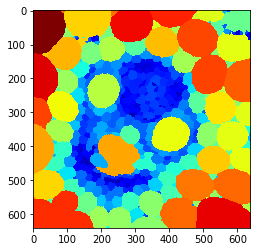# Quantitative maps from neighbor statistics#

import pyclesperanto_prototype as cle
import numpy as np
from numpy import random
import matplotlib


The example imge “maize_clsm.tif” was taken from the repository mathematical_morphology_with_MorphoLibJ and is licensed by David Legland under CC-BY 4.0 license

intensity_image = imread('../../data/maize_clsm.tif')
cle.imshow(intensity_image)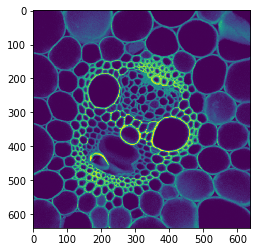# Starting point: Label map#

binary = cle.binary_not(cle.threshold_otsu(intensity_image))
cells = cle.voronoi_labeling(binary)

cle.imshow(cells, labels=True)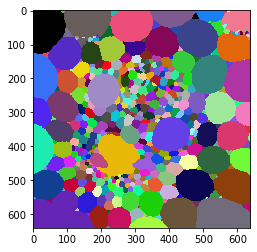## Nearest neighbor distance maps#

average_distance_of_n_closest_neighbors_map = cle.average_distance_of_n_closest_neighbors_map(cells, n=1)
cle.imshow(average_distance_of_n_closest_neighbors_map, color_map='jet')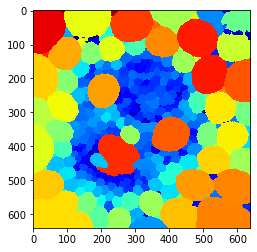average_distance_of_n_closest_neighbors_map = cle.average_distance_of_n_closest_neighbors_map(cells, n=5)
cle.imshow(average_distance_of_n_closest_neighbors_map, color_map='jet')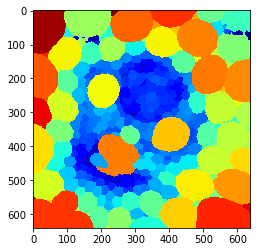## Touching neighbor distance map#

average_neighbor_distance_map = cle.average_neighbor_distance_map(cells)
cle.imshow(average_neighbor_distance_map, color_map='jet')